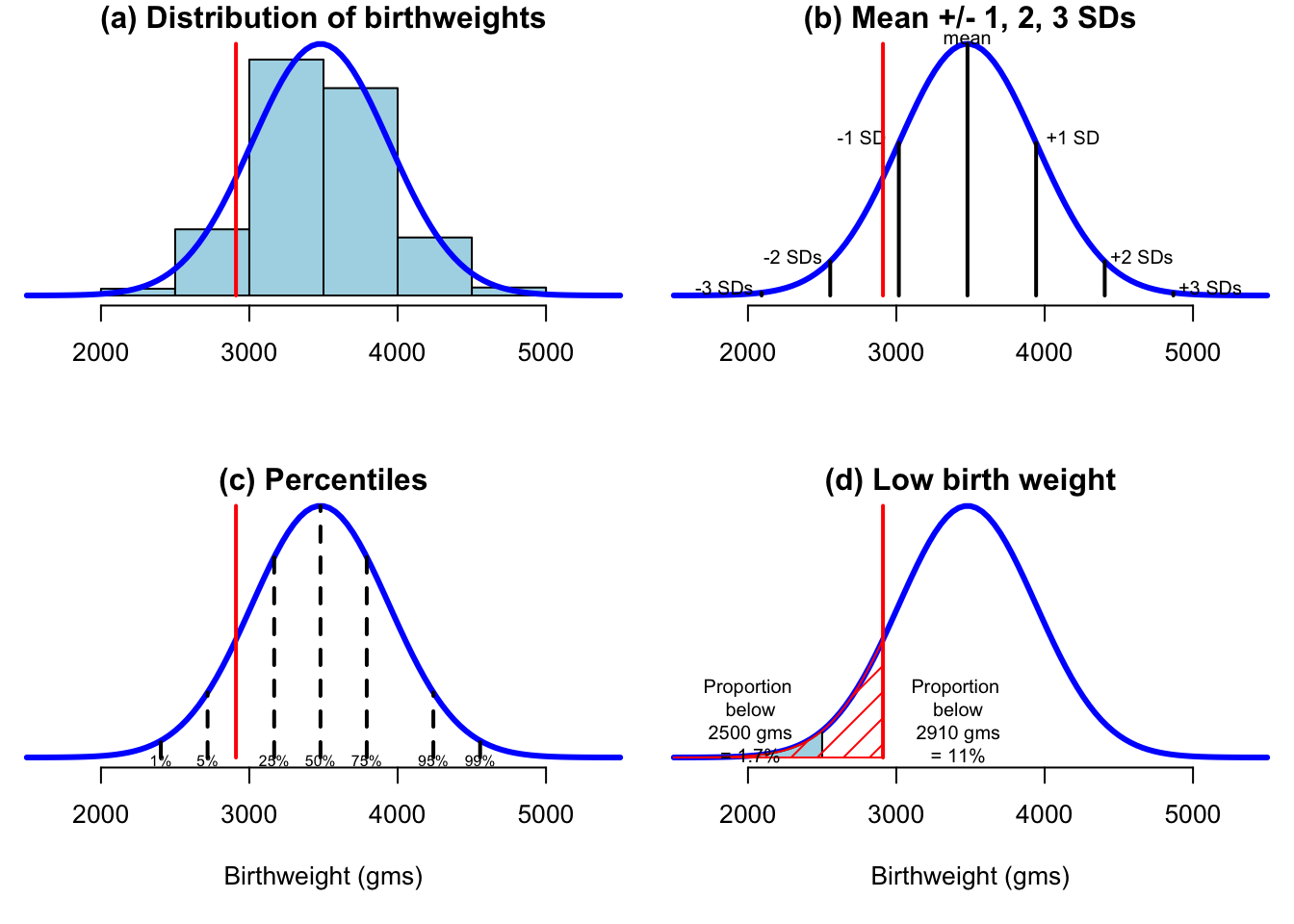The data are for 1,096,277 full-term births to non-hispanic whte women in the United States for 2013, and are taken from Table 23, page 51 of http://www.cdc.gov/nchs/data/nvsr/nvsr64/nvsr64_01.pdf,

weights=c(1500, 2000, 2500, 3000, 3500, 4000, 4500,5000)
mids=weights+250
n=c(5+48+308+1130, 12679, 124209, 442891, 389275, 108886, 14936,1345) # numbers in each bin
N=sum(n)  # total number of babies
area=N*500  # number * binwidth = total area of histogram

lbw   = sum(n[1:2])   # number with low birth weight (less than 2500)
lbw.percent=100*lbw/N  # % low birth weight
# 1.3%

#calculate mean and sd of population
# could use sheppard's correction

birth.mean=sum(n*mids/N)
birth.sd=sqrt( sum(n*(mids-birth.mean)^2)/N)

# per cent less than 2500 from normal approximation
lbw.est = 100 * pnorm(2500,birth.mean, birth.sd)
# 1.7%, good approxmation

#25th and 75th percentiles of population
qnorm(0.25, birth.mean,birth.sd)
##  3167.585
qnorm(0.75, birth.mean,birth.sd)
##  3791.655
# percentile of baby weighing 2910
xw = 2910
pnorm(xw, birth.mean,birth.sd)
##  0.1091087
par(mfrow=c(2,2))
# setup plot ranges noting max of normal density is at mean
xrange <- c(1500,5500)
yrange <- range( c(n, area*dnorm(birth.mean, birth.mean, birth.sd), 0))
scale=0.6
par(mar=c(5,0,1,0)+0.1)

# (a) empirical distribution and fitted normal
plot(xrange, yrange, type = "n", xlab = "", ylab = "",
bty="n",axes=F,main="(a) Distribution of birthweights", cex=scale)
axis(1,cex=scale)
# draw bars using rect and density using curve
rect(weights, 0, weights + 500, n, col = "lightblue")
curve(area*dnorm(x, birth.mean, birth.sd), min(xrange), max(xrange), add = TRUE,
lwd=3, col="blue")
lines(c(xw,xw),yrange,col="red",lwd=2)

# (b)   plot with sds
plot(xrange, yrange, type = "n", xlab = "", ylab = "",
bty="n",axes=F,,main="(b) Mean +/- 1, 2, 3 SDs" )
axis(1)
curve(area*dnorm(x, birth.mean, birth.sd), min(xrange), max(xrange), add = TRUE, lwd=3, col="blue")
I=-3:3
x1=birth.mean+I*birth.sd
y1=area*dnorm(x1,birth.mean, birth.sd)
label=c("-3 SDs", "-2 SDs", "-1 SD", "mean", "+1 SD","+2 SDs", "+3 SDs")
bit=10000
xx=250
shift=c(-xx,-xx,-xx,0,xx,xx,xx)
for(i in 1:7){
lines(c(x1[i],x1[i]), c(0,y1[i]),lwd=2)
text(x1[i]+shift[i],y1[i]+bit,label[i],cex=0.75)
}
lines(c(xw,xw),yrange,col="red",lwd=2)

# (c)  Percentiles
plot(xrange, yrange, type = "n", xlab = "Birthweight (gms)", ylab = "",
bty="n",axes=F,,main="(c) Percentiles" )
axis(1)
curve(area*dnorm(x, birth.mean, birth.sd), min(xrange), max(xrange), add = TRUE,
lwd=3, col="blue")
I=c(1,5,25,50,75,95,99)
x1=qnorm(I/100, birth.mean,birth.sd)
y1=area*dnorm(x1,birth.mean, birth.sd)
label=c("1%", "5%", "25%", "50%","75%","95%","99%")
bit=5000
for(i in 1:7){
lines(c(x1[i],x1[i]), c(0,y1[i]),lwd=2,lty=2)
text(x1[i],-bit,label[i],cex=0.6)
}
lines(c(xw,xw),yrange,col="red",lwd=2)

# (d)  Low birth weight
plot(xrange, yrange, type = "n", xlab = "Birthweight (gms)", ylab = "",
bty="n",axes=F,,main="(d) Low birth weight" )
axis(1)
curve(area*dnorm(x, birth.mean, birth.sd), min(xrange), max(xrange), add = TRUE,
lwd=3, col="blue")
x1=seq(1500,2500,10)
y1=area*dnorm(x1,birth.mean, birth.sd)
polygon(c(x1,x1[101:1]),c(rep(0,101), y1[101:1]),col="lightblue")

lines(c(xw,xw),yrange,col="red",lwd=2)
x1=seq(1500,xw,10)
nx=length(x1)
y1=area*dnorm(x1,birth.mean, birth.sd)
polygon(c(x1,x1[nx:1]),c(rep(0,nx), y1[nx:1]),col="red",density=10)

text(2000,70000,"Proportion\n below\n 2500 gms\n = 1.7%",cex=0.75)
text(3400,70000,"Proportion\n below\n 2910 gms\n = 11%",cex=0.75)Figure 3.2 (a) The distribution of birth weights of 1,096,277 children of non-Hispanic white women in the US in 2013, born at 39-40 weeks’ gestation, with a normal curve with the same mean and standard deviation as the recording weights in the population. A baby weighing 2,910g is shown as the dashed line. (b) The mean ?1,2,3 standard deviations (SDs) for the normal curve. (c) Percentiles of the normal curve. (d) The proportion of low-birth-weight babies (dark shaded area), and babies less than 2,910g (light shaded area).